Courses

# Test: APP And MRP (Level - 1)

## 20 Questions MCQ Test Manufacturing and Industrial Engineering | Test: APP And MRP (Level - 1)

Description
This mock test of Test: APP And MRP (Level - 1) for Mechanical Engineering helps you for every Mechanical Engineering entrance exam. This contains 20 Multiple Choice Questions for Mechanical Engineering Test: APP And MRP (Level - 1) (mcq) to study with solutions a complete question bank. The solved questions answers in this Test: APP And MRP (Level - 1) quiz give you a good mix of easy questions and tough questions. Mechanical Engineering students definitely take this Test: APP And MRP (Level - 1) exercise for a better result in the exam. You can find other Test: APP And MRP (Level - 1) extra questions, long questions & short questions for Mechanical Engineering on EduRev as well by searching above.
QUESTION: 1

Solution:
QUESTION: 2

Solution:
QUESTION: 3

### Planned order release (POR) is associated with

Solution:
QUESTION: 4

APP refers to

Solution:
QUESTION: 5

Time bucket is the term associated with

Solution:

The time period used for planning purposes in MRP-usually a week.

QUESTION: 6

Which of the following is not an APP strategy?

Solution:
QUESTION: 7

Which of the following is a constraint in preparing MPS?

Solution:
QUESTION: 8

The break-even point represents

Solution:
QUESTION: 9

Break-even analysis consists of

Solution:
QUESTION: 10

Break-down analysis shows profit when

Solution:
QUESTION: 11

Match List-I (Files in MRP) with List-II (Inputs required) and select the correct answer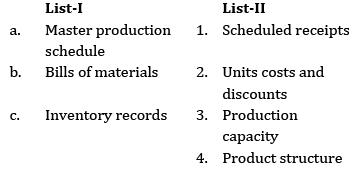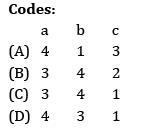Solution:
QUESTION: 12

Match the following: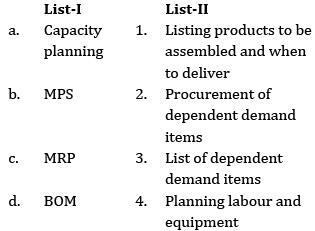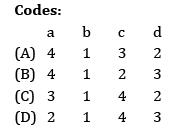Solution:
QUESTION: 13

Which of the following is a mixed strategy for APP?

Ans.:C

Solution:
QUESTION: 14

Which of the following is not a mixed strategy?

Solution:
QUESTION: 15

Which of the following is not included in inventory status file?

Ans~ D

Solution:
QUESTION: 16

Which of the following is not an output of MRP?

Solution:
QUESTION: 17

1. Flexible in nature

2. Easy to understand

3. Detailed operation can be visualized

Which of the above are the advantages of MPS?

Solution:
*Answer can only contain numeric values
QUESTION: 18

In a given product structure find the number of ‘B’ required to manufacture 100 ‘A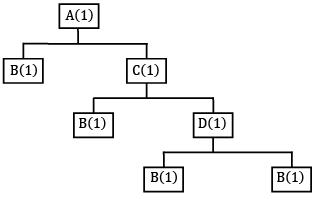Solution:

Total units of ‘B’ required to make one unit of A

= 1 × 1 + 1 × 1 × 1 + 1 × 1 × 1 × 1 + 1 × 1× 1 × 1 = 4

∴ Total units of ′ B′ required to manufacture 100 units of A = 4 × 100 = 400

QUESTION: 19

Consider the following data, for BEA: (BreakEven Analysis)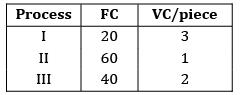If batch size is 100, then which is the most economic process?

Solution:

TC = FC + VC × Q

TC)I = 20 + 3 × 100 = 320

TC)II = 60 + 1 × 100 = 160

TC)III = 40 + 2 × 100 = 240

QUESTION: 20

The following data is related to a manufacturing company: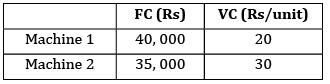What is BEV?

Solution:

(FC + VC × Q)1 = (FC + VC × Q)2

40,000 + 20 × Q = 35,000 + 30 × Q

5000 = 10 Q

Q = 500